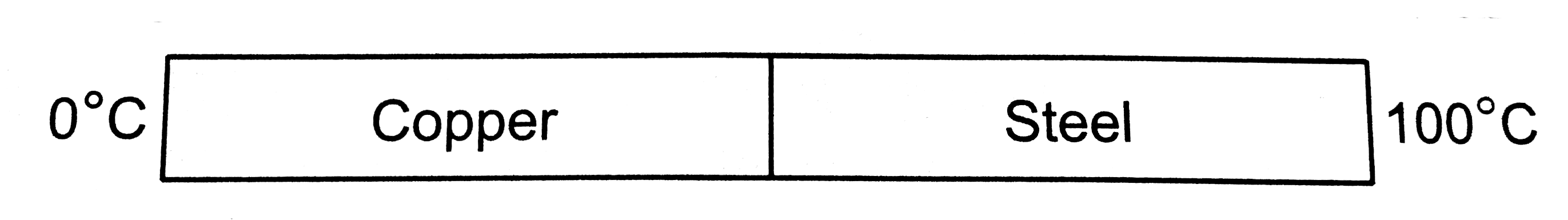# Figure shows a copper rod joined to a steel rod. The rods have equal length and and the equal cross sectional area. The free end of the copper rod is

62 views
in Physics
closed
Figure shows a copper rod joined to a steel rod. The rods have equal length and and the equal cross sectional area. The free end of the copper rod is kept at 0^(@)C and that of the steel rod is kept at 100^(@)C . Find the temperature at the junction of the rods. conductivity of copper =390WM^(-1).^(@)C^(-1) and that of steel =46Wm^(-1).^(@)C^(-1) .A. will be nore than 50^(@)C
B. will be less than 50^(@)C
C. will b 50^(@)C
D. may be nore or less than 50 c depending upon the size of rods

by (90.7k points)
selected

K_(C) gt K_(B)
therefore R_(C) lt R_(B)
Temperature difference across copper = HR_(C) = (TD)_(C)
And temperature difference across brass = HR_(B) = (TD)_(B)
Heat current H will be same in both , as they are in series.
Since R_(C) lt R_(B)
therefore (TD)_(c) lt (TD)_(B)
Or temperature of junction is more than 50^(@)C.